# K3-surface

Jump to: navigation, search

2010 Mathematics Subject Classification: Primary: 14J28 [MSN][ZBL]

A smooth projective algebraic surfacefor which the canonical class is trivial and for which the dimension,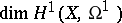, of the space of one-dimensional differential forms onis 0. The values of the following invariants for a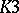-surface are known: the geometric genus,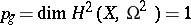, the Euler characteristic of the structure sheaf,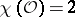, the étale or (over the field of complex numbers) the topological Betti numbers (cf. Betti number),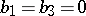and, and the Euler–Poincaré characteristic,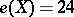. The Riemann–Roch formula for a one-dimensional invertible sheafon a-surface takes the formwhere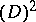is the index of self-intersection for the class of divisors corresponding to(see Riemann–Roch theorem). Ifcorresponds to an effective irreducible divisor, then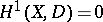.

The formula for the arithmetic genus of an irreducible curveonalso has a simple form: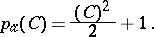As a result one obtains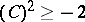, with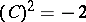only for smooth rational curves. It also follows thatis an even number for any divisor. Letbe the Néron–Severi group of the surface, i.e. the group of divisor classes onwith respect to algebraic equivalence. Then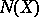is a free Abelian group of rank, whereif the characteristic of the ground fieldis 0, and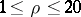or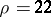if. The intersection index defines an integer-valued bilinear form onfor which the square of any element is even. Surfaces with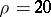(for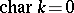) are called singular, while those with(for) are called supersingular.

Another numerical invariant for a surfaceis the minimal possible indexof self-intersection of an effective very ample divisor on, i.e. the minimum possible degree of a polarization on. If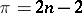, the surfacecan be imbedded in-dimensional projective space and cannot be imbedded in a projective space of lower dimension.

An important tool in studying-surfaces is to represent them as families (pencils) of elliptic curves. A surfaceis represented as a family of elliptic curves if one is given a regular mapping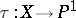all fibres of which, apart from a finite number, are non-singular elliptic curves. A surfacecan be represented in that form if and only if the groupcontains a non-zero element with self-intersection index 0, where all such representations correspond to the classes of effective divisors with self-intersection index 0. If a surface represented as a family of elliptic curves is a-surface, it does not have multiple fibres. The Jacobi elliptic family constructed from such a family is again a-surface.

An important class of-surfaces is provided by the Kummer surfaces. A Kummer surface is a non-singular model for the quotient of a two-dimensional Abelian varietywith respect to the automorphism subgroup generated by the sign-change mapping. In particular, the surface given by the equation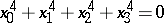in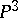is a Kummer surface. Any smooth surface of order four inis a-surface. A smooth surface obtained as the intersection of three hypersurfaces of order two (quadrics) inand a double covering oframified in a smooth curve of order six are-surfaces.

All-surfaces over the field of complex numbers are diffeomorphic; their moduli variety is connected and has dimension 19. One examines the structure of this variety and of-surface automorphisms by means of the period mapping. The period mapping for a-surface over the field of complex numbers is bijective (a theorem of Torelli type) .

If one is given a one-dimensional family of-surfaces (over) with one singular fibre, then the surface can be rearranged after covering the base, without alterations outside the singular fibre, so that that fibre either becomes non-singular or else is one of two types: a) the components of the singular fibre and the intersection curves are rational, and the dual polyhedron for the singular fibre has the topological type of a two-dimensional sphere; or b) the components of the singular fibre constitute a chain, and non-empty intersections occur only for adjacent surfaces, while the two extreme surfaces are rational surfaces, the middle ones are elliptic ruled surfaces and the intersection curves are elliptic. Types a) or b) arise when the monodromy of the family is non-trivial .-surfaces over an algebraically closed field of positive characteristic allow of a lifting into characteristic zero, their crystalline cohomology spaces do not have torsion, and their ranks coincide with the corresponding Betti numbers. For supersingular surfaces, an analogue of the period mapping has been constructed and a theorem of Torelli type has been proved. In this case the period variety is irreducible, complete, has dimension 9, and is unirational. All possible intersection forms onhave been described for supersingular surfaces, and there are nine of them for each value of the characteristic of the ground field .